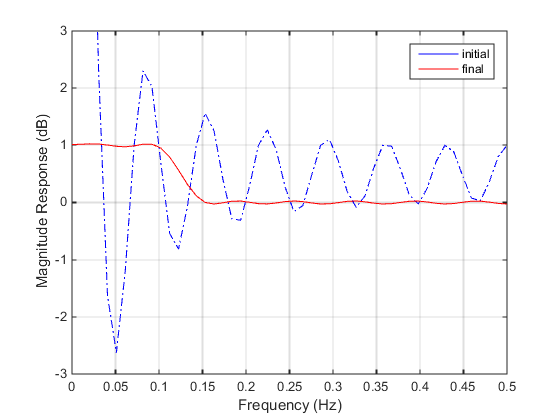## Signal Processing Using `fgoalattain`

Consider designing a linear-phase Finite Impulse Response (FIR) filter. The problem is to design a lowpass filter with magnitude one at all frequencies between 0 and 0.1 Hz and magnitude zero between 0.15 and 0.5 Hz.

The frequency response H(f) for such a filter is defined by

 $\begin{array}{c}H\left(f\right)=\sum _{n=0}^{2M}h\left(n\right){e}^{-j2\pi fn}\\ =A\left(f\right){e}^{-j2\pi fM},\\ A\left(f\right)=\sum _{n=0}^{M-1}a\left(n\right)\mathrm{cos}\left(2\pi fn\right),\end{array}$ (1)

where A(f) is the magnitude of the frequency response. One solution is to apply a goal attainment method to the magnitude of the frequency response. Given a function that computes the magnitude, `fgoalattain` will attempt to vary the magnitude coefficients a(n) until the magnitude response matches the desired response within some tolerance. The function that computes the magnitude response is given in `filtmin.m`. This function uses `a`, the magnitude function coefficients, and `w`, the discretization of the frequency domain of interest.

To set up a goal attainment problem, you must specify the `goal` and `weights` for the problem. For frequencies between 0 and 0.1, the goal is one. For frequencies between 0.15 and 0.5, the goal is zero. Frequencies between 0.1 and 0.15 are not specified, so no goals or weights are needed in this range.

This information is stored in the variable `goal` passed to `fgoalattain`. The length of `goal` is the same as the length returned by the function `filtmin`. So that the goals are equally satisfied, usually `weight` would be set to `abs(goal)`. However, since some of the goals are zero, the effect of using `weight=abs(goal)` will force the objectives with `weight` 0 to be satisfied as hard constraints, and the objectives with `weight` 1 possibly to be underattained (see Goal Attainment Method). Because all the goals are close in magnitude, using a `weight` of unity for all goals will give them equal priority. (Using `abs(goal)` for the weights is more important when the magnitude of `goal` differs more significantly.) Also, setting

`options = optimoptions('fgoalattain','EqualityGoalCount',length(goal)); `

specifies that each objective should be as near as possible to its goal value (neither greater nor less than).

### Step 1: Write a file filtmin.m

```function y = filtmin(a,w) n = length(a); y = cos(w'*(0:n-1)*2*pi)*a ;```

### Step 2: Invoke optimization routine

```% Plot with initial coefficients a0 = ones(15,1); incr = 50; w = linspace(0,0.5,incr); y0 = filtmin(a0,w); clf, plot(w,y0,'-.b'); drawnow; % Set up the goal attainment problem w1 = linspace(0,0.1,incr) ; w2 = linspace(0.15,0.5,incr); w0 = [w1 w2]; goal = [1.0*ones(1,length(w1)) zeros(1,length(w2))]; weight = ones(size(goal)); % Call fgoalattain options = optimoptions('fgoalattain','EqualityGoalCount',length(goal)); [a,fval,attainfactor,exitflag]=fgoalattain(@(x)filtmin(x,w0),... a0,goal,weight,[],[],[],[],[],[],[],options); % Plot with the optimized (final) coefficients y = filtmin(a,w); hold on, plot(w,y,'r') axis([0 0.5 -3 3]) xlabel('Frequency (Hz)') ylabel('Magnitude Response (dB)') legend('initial', 'final') grid on```

Compare the magnitude response computed with the initial coefficients and the final coefficients (Magnitude Response with Initial and Final Magnitude Coefficients). Note that you could use the `firpm` function in Signal Processing Toolbox™ software to design this filter.

Magnitude Response with Initial and Final Magnitude Coefficients